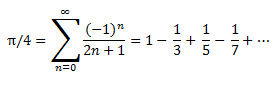In honor of National Pi Day, we thought it would be fun to calculate Pi and talk about performance differences between C# and C++. There is some math coming your way (yeah Math!), but feel free to skip to the end for a discussion on performance.

So back in the day, when things where WAY different, Pi was calculated using a mathematical series. One of the more famous ones uses the arctan math function to calculate the value of π. This is called the Leibniz formula for π and is represented byNow, this is one of the more inefficient ways to calculate π, it takes about 10 million terms to get an accuracy of 7 decimal places. For those of us asking performance differences between languages though, that is great!

We have written two programs to calculate π, one in C# and one in C++. They are both syntactically similar and use the same algorithm. We tried to get as close to an apples comparison as we could, but in comparing two different languages, we did our best, you are still comparing an apple to an orange.

So, what did we see? We calculated π to 15 decimal points, 3.141592653589793 for those of you keeping track at home. Both programs took almost 100 million iterations to converge, 99,995,330 to be exact. We ran each of the programs 10 times to get an average as shown in the following table

 Run C++ C# 1 4.384 6.908 2 4.299 6.466 3 4.3375 6.805 4 4.18 6.584 5 4.523 6.516 6 4.186 6.518 7 4.164 6.492 8 4.175 6.351 9 4.274 6.56 10 4.342 6.589 Average 4.29 6.58

The calculation of π using C++ is 35% faster that it's corresponding C# code. This is to be expected. Many modern languages such as C# and Java run through a managed runtime. This brings MANY efficiencies to developers. However, the runtime tends to add overhead when it comes to performance.

The pragmatic folks reading this realize, that the benefits of a managed execution environment and modern language generally outweigh performance gains from a language like C++. There are reasons that C# and Java are used instead of C++ because developers can be more productive. Our example here, using a very slow numerical algorithm and running it for ten million iterations is a stretch for most scenarios. The 2.5 second difference for such an intensive calculation, while relatively large in our example, in the grand scheme of things is not that big of difference.

So why bring it up? It is important to think through performance more and more in today's computing environment for two reasons. The first is cost. As more and more organization move to utility based computing with hosted services, such as Amazon Web Services (AWS) and Azure, organization are paying for their compute cost. If you can realize performance gains that reduce CPU cycles that has a direct impact on the bottom line. The second reason is that mobile is taking over the world, and mobile users want more battery life, not less. Fewer CPU cycles on a smartphone or tablet will result in better battery life.

It will be very rare that most organizations would use C++ or other "closer to the metal" languages for their Line of Business (LOB) applications. The moral of the story though is for intensive processes in cloud hosted or mobile environments, you want to be razor focused on optimizing your code for performance, no matter what language you choose.

Both code examples are included, so for National Pi day, make a circle, calculate some π, eat some pie and enjoy!

C++

``````// LifeOfPi.cpp : Defines the entry point for the console application.
//

#include "stdafx.h"
#include <iostream>
#include <iomanip>
using namespace std;

void pi( double *pi_value, int *iterations);

int main ( void )
{

int iterations = 0;     // number of actual loop iterations in pi
double pi_value = 0;    // computed value of pi

clock_t cBegin = (double)clock () ;
pi(&pi_value, &iterations);
printf("   %0.15f with %d iterations\n",  pi_value, iterations);
clock_t cEnd = (double) clock() ;

std::cout << float( cEnd - cBegin )

return 0;

}

void pi( double *pi_value, int *iterations)
{
int i;
int k=1;
double realPi = 3.141592653589793;
double epsilon = 0.00000001;
double delta=10;
*pi_value= 0;
while (delta>epsilon) {
*pi_value += pow(-1,(k+1))/(2*k-1);
delta = fabs((4* *pi_value)-realPi);
k++;
}
*iterations = k;
*pi_value = 4 * *pi_value;

return; // indicate program ended sucessfully

} // end fucntion main
``````

C#

``````using System;
using System.Collections.Generic;
using System.Linq;
using System.Text;

namespace LifeOfPiCSharp
{
class Program
{
static void Main(string[] args)
{
int iterations = 0;
double pi_value = 0;

DateTime startTime = DateTime.Now;
pi(out pi_value,out iterations);
DateTime endTime = DateTime.Now;
Console.WriteLine(pi_value.ToString() + " in " + iterations);
TimeSpan diff = endTime.Subtract(startTime);
Console.WriteLine(diff.Seconds + "."+diff.Milliseconds);
}

public static void pi(out double pi_value, out int totalIterations)
{
double realPi = 3.141592653589793;
double epsilon = 0.00000001;
double delta = 10;
int k = 1;

pi_value = 0;
while (delta > epsilon)
{
pi_value += Math.Pow(-1, (k + 1)) / (2 * k - 1);
delta = Math.Abs((4 * pi_value) - realPi);
k++;
}
totalIterations = k;
pi_value = 4 * pi_value;

}
}
}
``````

This blog post originally appeared at Skyline Technologies